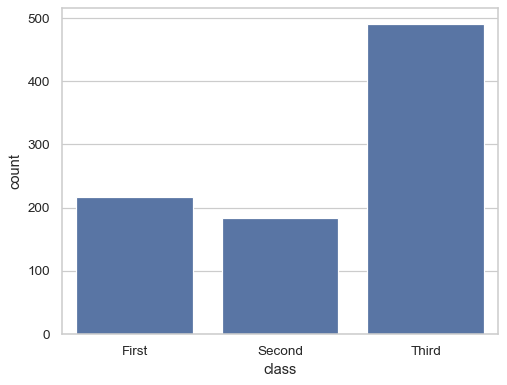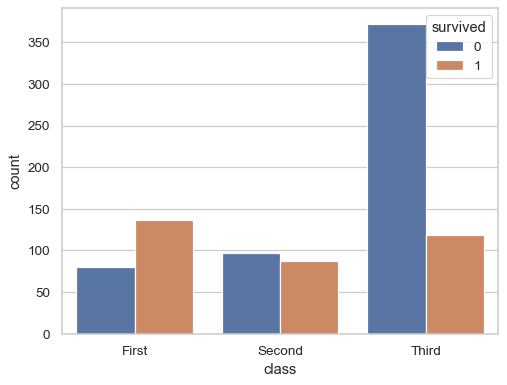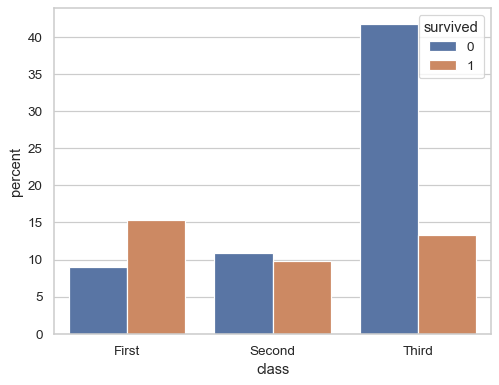# seaborn.countplot#

seaborn.countplot(data=None, *, x=None, y=None, hue=None, order=None, hue_order=None, orient=None, color=None, palette=None, saturation=0.75, fill=True, hue_norm=None, stat='count', width=0.8, dodge='auto', gap=0, log_scale=None, native_scale=False, formatter=None, legend='auto', ax=None, **kwargs)#

Show the counts of observations in each categorical bin using bars.

A count plot can be thought of as a histogram across a categorical, instead of quantitative, variable. The basic API and options are identical to those for `barplot()`, so you can compare counts across nested variables.

Note that `histplot()` function offers similar functionality with additional features (e.g. bar stacking), although its default behavior is somewhat different.

Note

By default, this function treats one of the variables as categorical and draws data at ordinal positions (0, 1, … n) on the relevant axis. As of version 0.13.0, this can be disabled by setting `native_scale=True`.

Parameters:
dataDataFrame, Series, dict, array, or list of arrays

Dataset for plotting. If `x` and `y` are absent, this is interpreted as wide-form. Otherwise it is expected to be long-form.

x, y, huenames of variables in `data` or vector data

Inputs for plotting long-form data. See examples for interpretation.

order, hue_orderlists of strings

Order to plot the categorical levels in; otherwise the levels are inferred from the data objects.

orient“v” | “h” | “x” | “y”

Orientation of the plot (vertical or horizontal). This is usually inferred based on the type of the input variables, but it can be used to resolve ambiguity when both `x` and `y` are numeric or when plotting wide-form data.

Changed in version v0.13.0: Added ‘x’/’y’ as options, equivalent to ‘v’/’h’.

colormatplotlib color

Single color for the elements in the plot.

palettepalette name, list, or dict

Colors to use for the different levels of the `hue` variable. Should be something that can be interpreted by `color_palette()`, or a dictionary mapping hue levels to matplotlib colors.

saturationfloat

Proportion of the original saturation to draw fill colors in. Large patches often look better with desaturated colors, but set this to `1` if you want the colors to perfectly match the input values.

hue_normtuple or `matplotlib.colors.Normalize` object

Normalization in data units for colormap applied to the `hue` variable when it is numeric. Not relevant if `hue` is categorical.

New in version v0.12.0.

stat{‘count’, ‘percent’, ‘proportion’, ‘probability’}

Statistic to compute; when not `'count'`, bar heights will be normalized so that they sum to 100 (for `'percent'`) or 1 (otherwise) across the plot.

New in version v0.13.0.

widthfloat

Width allotted to each element on the orient axis. When `native_scale=True`, it is relative to the minimum distance between two values in the native scale.

dodge“auto” or bool

When hue mapping is used, whether elements should be narrowed and shifted along the orient axis to eliminate overlap. If `"auto"`, set to `True` when the orient variable is crossed with the categorical variable or `False` otherwise.

Changed in version 0.13.0: Added `"auto"` mode as a new default.

log_scalebool or number, or pair of bools or numbers

Set axis scale(s) to log. A single value sets the data axis for any numeric axes in the plot. A pair of values sets each axis independently. Numeric values are interpreted as the desired base (default 10). When `None` or `False`, seaborn defers to the existing Axes scale.

New in version v0.13.0.

native_scalebool

When True, numeric or datetime values on the categorical axis will maintain their original scaling rather than being converted to fixed indices.

New in version v0.13.0.

formattercallable

Function for converting categorical data into strings. Affects both grouping and tick labels.

New in version v0.13.0.

legend“auto”, “brief”, “full”, or False

How to draw the legend. If “brief”, numeric `hue` and `size` variables will be represented with a sample of evenly spaced values. If “full”, every group will get an entry in the legend. If “auto”, choose between brief or full representation based on number of levels. If `False`, no legend data is added and no legend is drawn.

New in version v0.13.0.

axmatplotlib Axes

Axes object to draw the plot onto, otherwise uses the current Axes.

kwargskey, value mappings

Other parameters are passed through to `matplotlib.patches.Rectangle`.

Returns:
axmatplotlib Axes

Returns the Axes object with the plot drawn onto it.

`histplot`

Bin and count observations with additional options.

`barplot`

Show point estimates and confidence intervals using bars.

`catplot`

Combine a categorical plot with a `FacetGrid`.

Examples

Show the count of value for a single categorical variable:

```sns.countplot(titanic, x="class")
```Group by a second variable:

```sns.countplot(titanic, x="class", hue="survived")
```Normalize the counts to show percentages:

```sns.countplot(titanic, x="class", hue="survived", stat="percent")
```